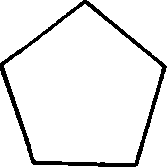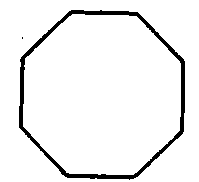A polygon is a plane figure bounded by straight lines. The boundary lines are called the sides and the sum of the sides is called the perimeter.

Polygons are classified according to the number of sides.

A triangle is a polygon of three sides.

A quadrilateral is a polygon of four sides. A pentagon is a polygon of five sides, Fig. 43. A hexagon is a polygon of six sides, Fig. 44. A heptagon is a polygon of seven sides. An octagon is a polygon of eight sides, Fig. 45.Fig. 43. Pentagon.Fig. 44. Hexagon.Fig. 45. Octagon.

A decagon is a polygon of ten sides.

A dodecagon is a polygon of twelve sides.

An equilateral polygon is one all of whose sides are equal.

An equiangular polygon is one all of whose angles are equal.

A regular polygon is one all of whose angles and all of whose sides are equal.

Triangles

A triangle is a polygon enclosed by three straight lines called sides. The angles of a triangle are the angles formed by the sides.

A right-angled triangle, often called a right triangle, Fig. 46, is one that has a right angle. The longest side (the one opposite the right angle) is called the hypotenuse, and the other sides are sometimes called legs.Fig. 46. Right-Angled Triangle.Fig. 47. Acute Angled Triangle.Fig. 48. Obtuse-Angled Triangle.

An acute-angled triangle is one that has all of its angles acute, Fig. 47.

An obtuse-angled triangle is one that has an obtuse angle, Fig. 48.

An equilateral triangle is one having all of its sides equal, Fig. 49.

An equiangular triangle is one having all of its angles equal.

An isosceles triangle, Fig. 50, is one, two of whose sides are equal. A scalene triangle, Fig. 51, is one, no two of whose sides are equal.Fig. 49. Equilateral Triangle.Fig. 50. Isosceles Triangle.Fig. 51. Scalene Triangle.

The base of a triangle is the lowest side; it is the side upon which the triangle is supposed to stand. Any side may, however, be taken as the base. In an isosceles triangle, the side which is not one of the equal sides is usually considered as the base.

The altitude of a triangle is the perpendicular drawn from the vertex to the base.

A quadrilateral is a polygon bounded by four straight lines, as Fig. 52.

The diagonal of a quadrilateral is a straight line joining two opposite vertices.

Trapezium

A trapezium is a quadrilateral, no two of whose sides are parallel.

Trapezoid

A trapezoid is a quadrilateral having two sides parallel, Fig. 53. The parallel sides are called the bases and the perpendicular distance between the bases is called the attitude.Fig. 53. Trapezoid.Fig. 54. Parallelogram.

Parallelogram

A parallelogram is a quadrilateral whose opposite sides are parallel, Fig. 54.

There are four kinds of parallelograms: rectangle, square, rhombus, and rhomboid.

The rectangle, Fig. 55, is a parallelogram whose angles are right angles.

The Square

Fig. 56, is a parallelogram all of whose sides are equal and whose angles are right angles.

The rhombus, Fig. 57, is a parallelogram whose sides are equal but whose angles are not right angles.Fig. 55. Rectangle.Fig. 56. Square.Fig. 57. Rhombus.

The rhomboid is a parallelogram whose adjacent sides are unequal, and whose angles are not right angles.